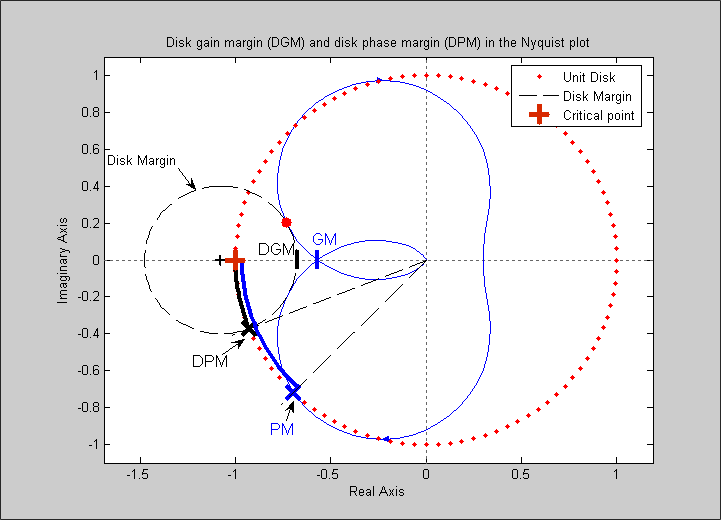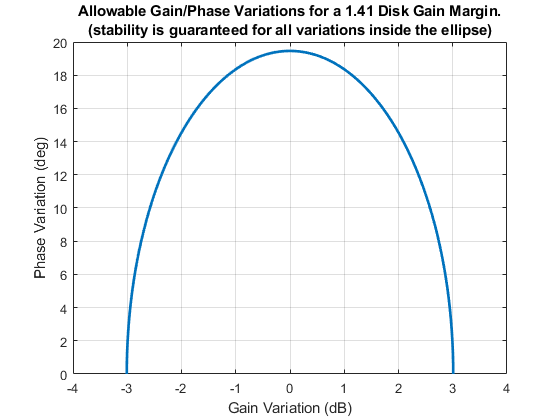# dmplot

(Not recommended) Interpret disk gain and phase margins

`dmplot` is not recommended. Use `diskmarginplot` instead.

## Syntax

```dmplot
dmplot(diskgm)
```

`[dgm,dpm] = dmplot`

## Description

`dmplot` brings up a plot that illustrates the disk gain margin (`dgm`) and disk phase margin (`dpm`) for a sample system. Both margins are derived from the largest disk that

• Contains the critical point (–1,0)

• Does not intersect the Nyquist plot of the open-loop response L

`diskgm` is the radius of this disk and a lower bound on the classical gain margin.

`dmplot(diskgm)` plots the maximum allowable phase variation as a function of the actual gain variation for a given disk gain margin `diskgm` (the maximum gain variation being `diskgm`). The closed-loop system is guaranteed to remain stable for all combined gain/phase variations inside the plotted ellipse.

`[dgm,dpm] = dmplot` returns the data used to plot the gain/phase variation ellipse.

## Examples

collapse all

When you call `dmplot` without an argument, the resulting plot and text shows a comparison of a disk margin analysis with the classical notations of gain and phase margins. The Nyquist plot is of the loop transfer function L(s):

`$L\left(s\right)=\frac{\frac{s}{30}+1}{\left(s+1\right)\left({s}^{2}+1.6s+16\right)}.$`

`dmplot`
``` This figure shows a comparison of a disk margin analysis with the classical notations of gain and phase margins. The Nyquist plot is of the loop transfer function L = 4(s/30 + 1)/((s+1)*(s^2 + 1.6s + 16)) - The Nyquist plot of L corresponds to the blue line - The unit disk corresponds to the dotted red line - GM and PM indicate the location of the classical gain and phase margins for the system L. - DGM and DPM correspond to the disk gain and phase margins. The disk margins provide a lower bound on classical gain and phase margins. - The disk margin circle corresponds to the dashed black line. The disk margin corresponds to the largest disk centered at (GMD + 1/GMD)/2 that just touches the loop transfer function L. This location is indicated by the red dot. ```The x-axis corresponds to the gain variation in dB and the y-axis corresponds to the allowable phase variation in degrees. For a disk gain margin corresponding to 3 dB (1.414), the closed-loop system is stable for all phase and gain variations inside the blue ellipse. For example, the closed-loop system can simultaneously tolerate +/– 2 dB gain variation and +/– 14 deg phase variations. To see the allowable variations for a given disk gain margin, use the given value as an input to `dmplot`.

```figure % new figure window dmplot(1.414)```## Compatibility Considerations

expand all

Not recommended starting in R2020a

## References

Barrett, M.F., Conservatism with robustness tests for linear feedback control systems, Ph.D. Thesis. Control Science and Dynamical Systems, University of Minnesota, 1980.

Blight, J.D., R.L. Dailey, and Gangsass, D., “Practical control law design for aircraft using multivariable techniques,” International Journal of Control, Vol. 59, No. 1, 1994, 93-137.

Bates, D., and I. Postlethwaite, Robust Multivariable Control of Aerospace Systems, Delft University Press, Delft, The Netherlands, ISBN: 90-407-2317-6, 2002.